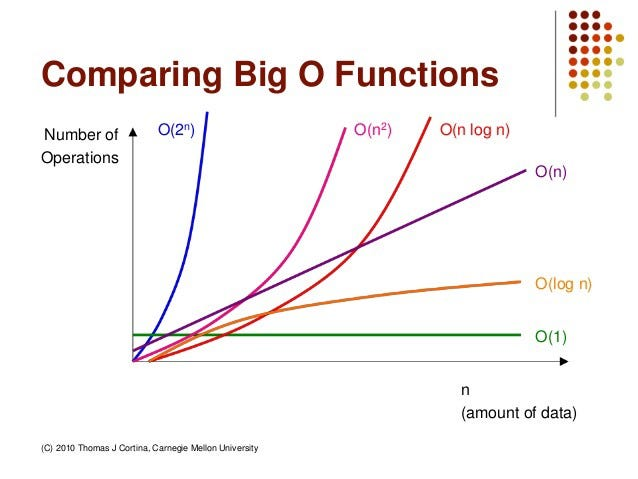Sorting Algorithms

First, a broad question. Why do we need sorting in the first place? The short answer is that it makes searching easier and faster.

Now before diving into sorting algorithms, let’s learn about time complexity. By time we don’t mean time in the traditional sense of seconds and minutes. We mean time in terms of steps.

Big Oh

It is relationship we use between the number of steps and the number of elements for our input size. It is basically the algorithm’s upper bound, the worst-case scenario, the maximum number of steps, running the complete algorithm will take.1X-axis: amount of data, y-axis: num of operations (aka how long it will take)

We care so much about time complexity because it makes comparing and classifying algorithms simpler and when we scale our algorithms, efficiency matters a lot.

Now, we’re going to learn about four sorting algorithms.

• Selection sort
• Insertion sort
• Merge sort

Bubble Sort

So one way I like to think about it. is imagine if you are going through an array and you have sort of like a circular lens, sort of your bubble and you’re comparing and sorting only 2 adjacent elements at a time. Another way I like to think about it is, we have sort of like a bubble monster that wants to organize these buckets of bubble treats with the biggest bucket closest to his house. And he can only carry one bucket at a time or else he’ll pop and you also can’t see very well so you can only compare between the two adjacent buckets.

begin BubbleSort(list)for all elements of list
if list[i] > list[i+1]
swap(list[i], list[i+1])
end if
end for

return list

end BubbleSort

It’s time complexity is O(n²).

Selection Sort

Conceptually this algorithm reminds me of the notion of triage and an emergency room. So I think of it sort of as a doctor that scans the room, finds the sickest person and says you go to the front of the line and then the next sickest person goes to the front of line and so forth. Here’s another way of thinking about this algorithm. Imagine there’s a little construction worker and he uses some binoculars and scans and finds the smallest element in the array and then brings it to the front and then the second smallest and then brings up to the second position in the line and so forth.

begin selectionSort(list, n)for i = 1 to n - 1
/* set current element as minimum*/
min = i

/* check the element to be minimum */
for j = i+1 to n
if list[j] < list[min] then
min = j;
end if
end for
/* swap the minimum element with the current element*/
if indexMin != i then
swap list[min] and list[i]
end if
end for

end procedure

It’s time complexity is O(n²).

Insertion Sort

Now I think this is a sorting algorithm that many of us use in our daily lives. So for instance right before a test I will often organize all of my math notes. And for the most part usually they’re all pretty sorted. Sometimes I’ll pull out one and then to put it back in to organize the whole pile, I just have to insert it in the right location. A pretty common way of thinking about the insertion sort algorithm, is to think about how you would sort cards. While the first card is always sorted and we go to the next card I mean you compare it to the first card and put it in the right place. Then you go to the third card and then figure out where it fits within the first two cards and then so on. This means that every step of the way you’re gonna have a part of your card pile sorted and a part that needs sorting.

begin insertionSort( A : array of items )
int holePosition
int valueToInsert

for i = 1 to length(A) inclusive do:

/* select value to be inserted */
valueToInsert = A[i]
holePosition = i

/*locate hole position for the element to be inserted */

while holePosition > 0 and A[holePosition-1] > valueToInsert do:
A[holePosition] = A[holePosition-1]
holePosition = holePosition -1
end while

/* insert the number at hole position */
A[holePosition] = valueToInsert

end for

end procedure

It’s time complexity is O(n²).

Merge Sort

This is our divide and conquer algorithm. So the way this algorithm works is, first the elements are. split up and then they are sorted and merged together in small chunks that are then worked together in bigger chunks and so forth.

begin mergesort( var a as array )
if ( n == 1 ) return a
var l1 as array = a ... a[n/2]
var l2 as array = a[n/2+1] ... a[n]
l1 = mergesort( l1 )
l2 = mergesort( l2 )
return merge( l1, l2 )
end procedure
begin merge( var a as array, var b as array )var c as array
while ( a and b have elements )
if ( a > b )
add b to the end of c
remove b from b
else
add a to the end of c
remove a from a
end if
end while

while ( a has elements )
add a to the end of c
remove a from a
end while

while ( b has elements )
add b to the end of c
remove b from b
end while

return c

end procedure

It’s time complexity is O(nlogn).

This article is meant to present you with an introduction to sorting algorithms. I encourage you to try implementing these algorithms on your own and explore more around the internet to learn more sorting algorithms. If this is interesting to you, I highly suggest watching some videos or using some of the many interactive, online tools available to hone your understanding.

So… *as mentioned in Finding Nemo* just keep sorting